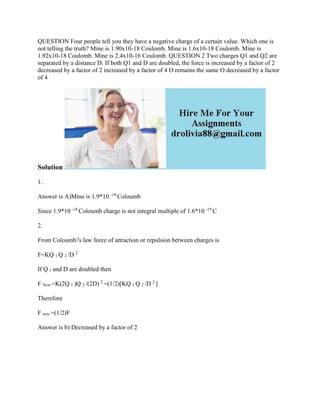Se está descargando tu SlideShare. ×

# QUESTION Four people tell you they have a negative charge of a certain.docx

Anuncio
Anuncio
Anuncio
Anuncio
Anuncio
Anuncio
Anuncio
Anuncio
Anuncio
Anuncio
AnuncioCargando en…3
×

1 de 2 Anuncio

# QUESTION Four people tell you they have a negative charge of a certain.docx

QUESTION Four people tell you they have a negative charge of a certain value. Which one is not telling the truth? Mine is 1.90x10-18 Coulomb. Mine is 1.6x10-18 Coulomb. Mine is 1.92x10-18 Coulomb. Mine is 2.4x10-16 Coulomb. QUESTION 2 Two charges Q1 and Q2 are separated by a distance D. If both Q1 and D are doubled, the force is increased by a factor of 2 decreased by a factor of 2 increased by a factor of 4 O remains the same O decreased by a factor of 4
Solution
1.
Answer is A)Mine is 1.9*10 -18 Coloumb
Since 1.9*10 -18 Coloumb charge is not integral multiple of 1.6*10 -19 C
2.
From Coloumb\'s law force of attraction or repulsion between charges is
F=KQ 1 Q 2 /D 2
If Q 1 and D are doubled then
F New =K(2Q 1 )Q 2 /(2D) 2 =(1/2)[KQ 1 Q 2 /D 2 ]
Therefore
F new =(1/2)F
Answer is b) Decreased by a factor of 2
.

QUESTION Four people tell you they have a negative charge of a certain value. Which one is not telling the truth? Mine is 1.90x10-18 Coulomb. Mine is 1.6x10-18 Coulomb. Mine is 1.92x10-18 Coulomb. Mine is 2.4x10-16 Coulomb. QUESTION 2 Two charges Q1 and Q2 are separated by a distance D. If both Q1 and D are doubled, the force is increased by a factor of 2 decreased by a factor of 2 increased by a factor of 4 O remains the same O decreased by a factor of 4
Solution
1.
Answer is A)Mine is 1.9*10 -18 Coloumb
Since 1.9*10 -18 Coloumb charge is not integral multiple of 1.6*10 -19 C
2.
From Coloumb\'s law force of attraction or repulsion between charges is
F=KQ 1 Q 2 /D 2
If Q 1 and D are doubled then
F New =K(2Q 1 )Q 2 /(2D) 2 =(1/2)[KQ 1 Q 2 /D 2 ]
Therefore
F new =(1/2)F
Answer is b) Decreased by a factor of 2
.

Anuncio
Anuncio

### QUESTION Four people tell you they have a negative charge of a certain.docx

1. 1. QUESTION Four people tell you they have a negative charge of a certain value. Which one is not telling the truth? Mine is 1.90x10-18 Coulomb. Mine is 1.6x10-18 Coulomb. Mine is 1.92x10-18 Coulomb. Mine is 2.4x10-16 Coulomb. QUESTION 2 Two charges Q1 and Q2 are separated by a distance D. If both Q1 and D are doubled, the force is increased by a factor of 2 decreased by a factor of 2 increased by a factor of 4 O remains the same O decreased by a factor of 4 Solution 1. Answer is A)Mine is 1.9*10 -18 Coloumb Since 1.9*10 -18 Coloumb charge is not integral multiple of 1.6*10 -19 C 2. From Coloumb's law force of attraction or repulsion between charges is F=KQ 1 Q 2 /D 2 If Q 1 and D are doubled then F New =K(2Q 1 )Q 2 /(2D) 2 =(1/2)[KQ 1 Q 2 /D 2 ] Therefore F new =(1/2)F Answer is b) Decreased by a factor of 2